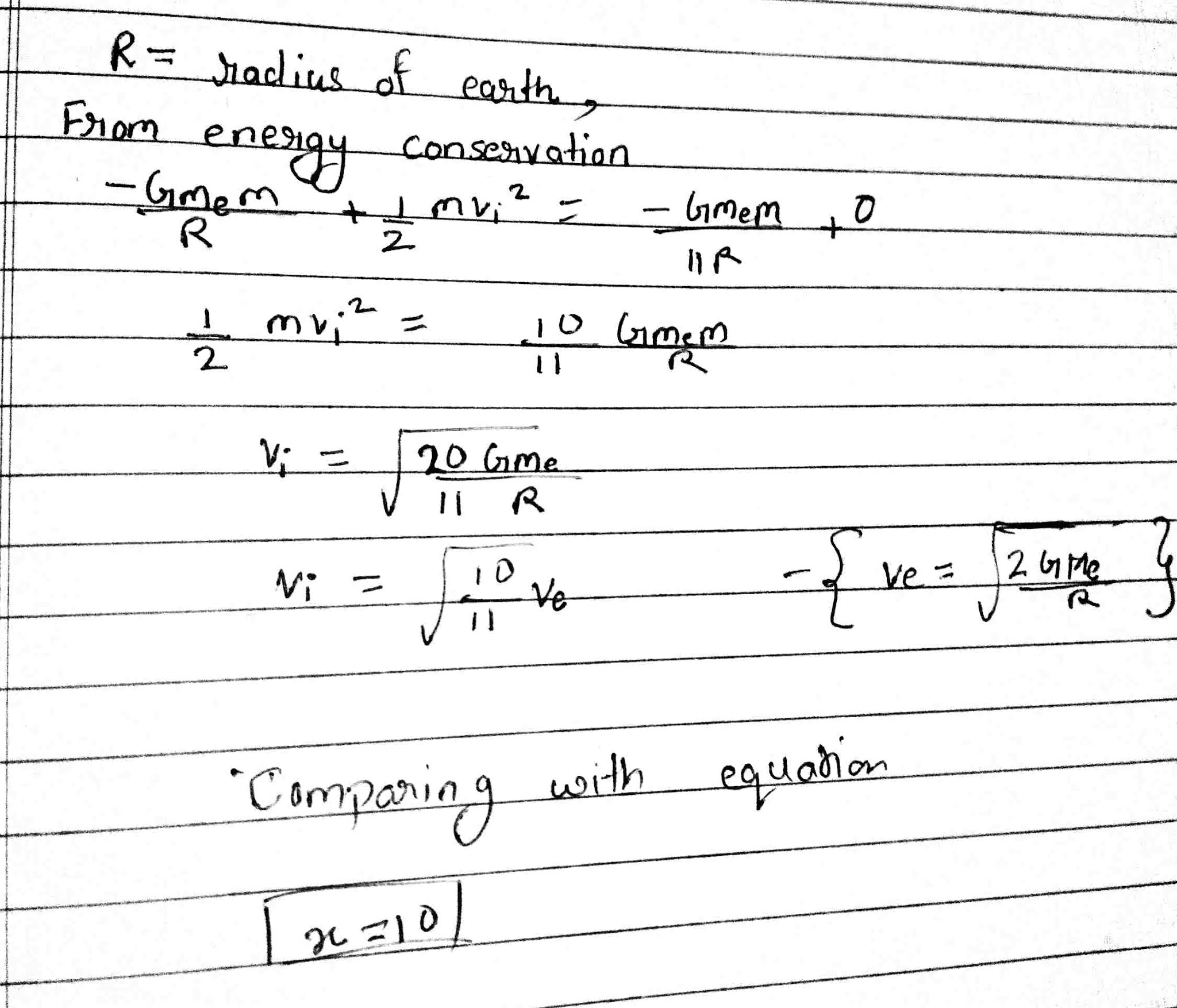# The initial velocity vi required to project a body vertically upward from the surface of the earth to reach a height of 10R, where R is the radius of the earth,

The initial velocity vi required to project a body vertically upward from the surface of the earth to reach a height of 10R, where R is the radius of the earth, may be described in terms of escape velocity ve such that  $$v_i=\sqrt{\frac{x}{y}}\times v_e$$.The value of x will be ________.

from jee-2021